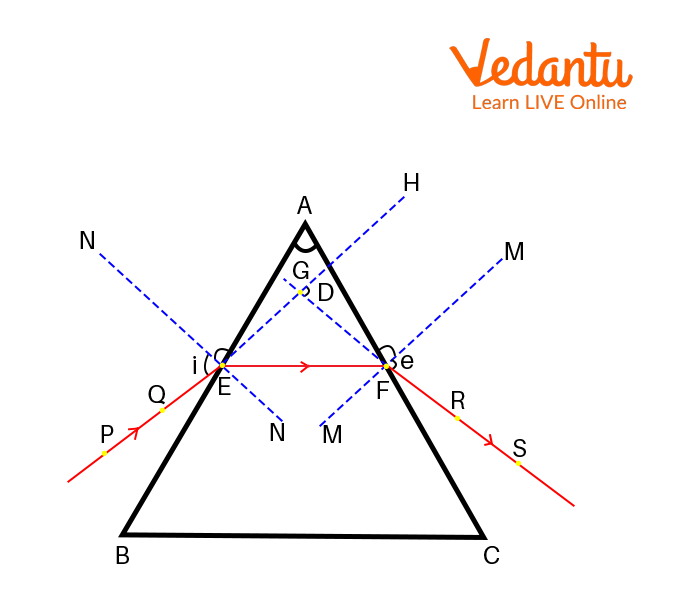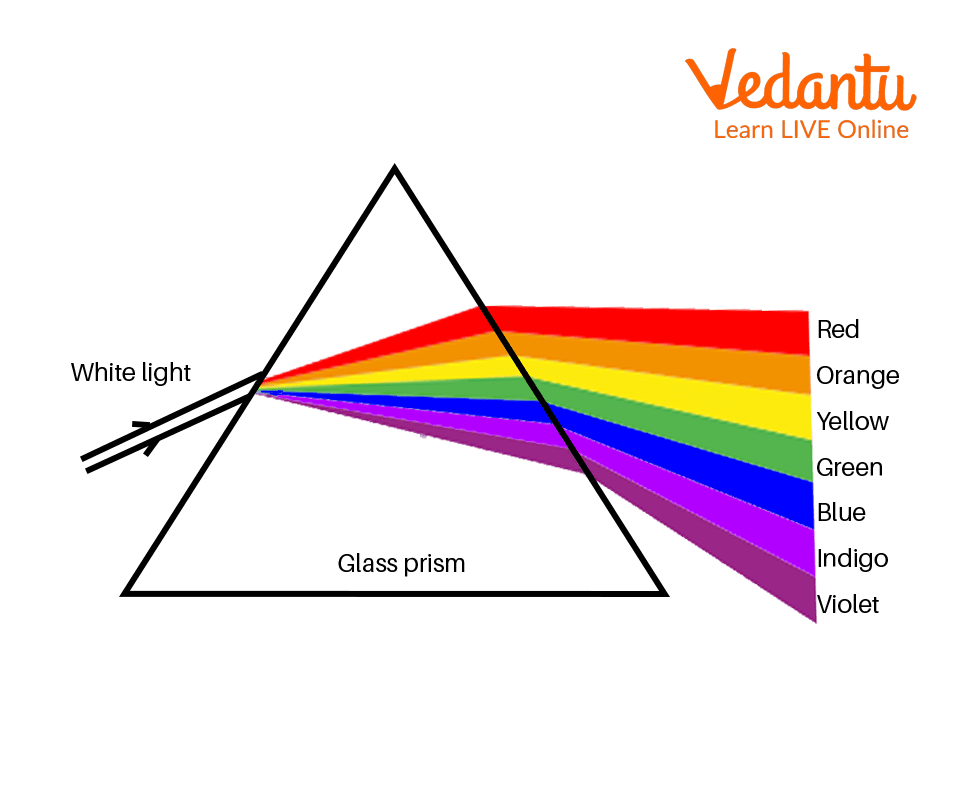Courses
Courses for Kids
Free study material
Free LIVE classes
More

# Refraction Through Prism - Principle and Working for JEE## What is the Refraction of Light?

Last updated date: 27th Mar 2023
Total views: 82.2k
Views today: 0.59k

Usually prisms can be made from transparent materials like glass, plastic, and fluorite. Generally, flint glass or crown glass can be used to make prisms.Triangular Glass Prism

Here, we have shown one example of a glass prism which is triangular in shape. Glass prisms are available in many shapes like rectangular glass prism, polygonal prisms. Here, we will see how these kinds of glass prisms play an important role to understand the phenomena of refraction and dispersion of light

In this article, we will have a deep insight into the concept of refraction of light, dispersion of light, and their uses in real life.

## Refraction of Light Through a Prism

Refraction of light is one of the most important phenomena in optics. Refraction of light is defined as a change in direction of light when it travels obliquely from one medium to another medium. When light ray travels from rarer to denser medium, it bends toward the normal and when it travels from denser to rarer medium, it bends away from the normal.

In the prism diagram given below, an incident ray PQ is incident on the first surface AB of a glass prism from air to glass medium. Here, light is travelling from a rarer medium to a denser medium. So, according to Snell’s law, the light ray on refraction bends towards the normal. Now, a light ray enters from glass to air medium at the other surface AC. Here, glass is denser than air, and thus while moving from glass to air, the emergent ray RS bends away from the normal.Refraction of Light through a Glass Prism

PQ = incident ray

EF = refracted ray

RS = emergent ray

$\angle i$ = angle of incidence

$\angle e$ = angle of emergence

$\angle A$ = angle of prism

$\angle D$ = angle of deviation

The angle of incidence is the angle made by line PE with the normal N. The angle of emergence is the angle made by the line SF with the normal M. The angle made by PG and SG is the angle of deviation. We observe from the figure that at the surface AB, the light ray enters and bends towards the normal on refraction and at the surface AC, the light ray bends away from the normal. In this way, the refraction of light takes place through a glass prism.

You can take a pencil and put that pencil in a glass of water. The pencil will appear bent. Pencil bends due to the optical phenomena of refraction of light. This is the best example to understand the concept of refraction of light. This concept of refraction of light is also used in cameras, binoculars, and other devices. Stars twinkle in the sky due to this concept.

## What is the Dispersion of Light?

Dispersion of light was first discovered by Sir Isaac Newton. When you incident white light on a glass prism, it splits white light into its constituent colours. So, we can say splitting of white light into its constituent colours is called dispersion of light and the band of colours obtained by the dispersion of light is called spectrum. The sequence of bands of colours will be violet, indigo, blue, green, yellow, orange, and red.

Light rays of different colours travel with the same speed in vacuum, but they travel with different speeds in different mediums. Red light has the maximum and violet has the minimum wavelength. Wavelength of light is directly proportional to velocity. So, red light travels fastest, while violet light travels slowest in any medium.

## Dispersion of Light by a Glass Prism

Dispersion of white light takes place because while passing through the transparent medium, the angle of refraction of lights of different colours is different. When white light incidents on the glass prism, it will experience refraction of light. Red colour has a maximum wavelength. So, it will deviate least and create the upper part of the spectrum. Violet has the least wavelength, so it deviates the most.Dispersion of Light by a Glass Prism

One simple example is the beautiful rainbow where sunlight splits into different colours which is an example of white light dispersion and another examples are the dispersion of colours in soap bubbles, dispersion of colours on the backside of a CD. Different colours at the backside of a CD almost look like a rainbow. These are the examples of dispersion of light.

## Rainbow Formation

A rainbow is generally formed when the Sun appears just after it has rained. At this time, many water droplets will be present in the air. When the sunlight incidents on these water droplets, dispersion of light takes place while undergoing refraction because these tiny water droplets act like small prisms. In this way, a beautiful rainbow is formed. Places where you can always see a natural rainbow are Niagara falls, Victoria falls, and The Canadian Rockies.

## Solved Examples

1. The refractive index of water is 1.33 and the speed of light in air is $3 \times {10^8}m{s^{ - 1}}$. Calculate the speed of light in water.

Ans: Given, the refractive index of water $n = 1.33$

$c = 3 \times {10^8}m{s^{ - 1}}$

The refractive index is given by $n = \frac{c}{v}$

Substituting the given values in the above equation, we will get $v = \frac{c}{n} = \frac{{3 \times {{10}^8}}}{{1.33}}$

$\therefore v = 2.25 \times {10^8}$

So, the speed of light in glass would be $2.25 \times {10^8}$.

## Interesting Facts

• When we pass red light through a glass prism, it will not split into different colours because it has a single wavelength.

• If we pass red colour light through a glass prism, then it will deviate least.

• A glass prism can produce spectrum but a glass slab can not produce it.

## Conclusion

In simple words, we can conclude that a glass prism is a solid, homogeneous, transparent, and refracting medium which is bounded by two plane surfaces inclined at an angle. A ray of white light gets dispersed when it passes through a glass prism.

## FAQs on Refraction Through Prism - Principle and Working for JEE

1. What will be the outcomes if there is no dispersion?

If the dispersion is not present in the earth, then the outcomes will be quite different. If the dispersion is not possible, then there will be no possibility of scattering light. If the scattering of light is not possible, then there will be no formation of the rainbow after the rain. There will be no splitting of white light when it passes through the glass prism. The colour of the wavelength cannot be determined if there is no scattering of light. Hence, there will be a worse outcome if there is no dispersion.

2. What is the basic reason for the process dispersion?

It is known that dispersion is the process of splitting up white light when it passes through the glass prism due to the refraction of light. The basic cause behind the process of dispersion is that light is made up of different colours having different wavelengths. When this white light is passed through the glass prism, every colour of a different wavelength deviates at certain angles depending upon the refraction through the glass prism. Hence, different colours are obtained from a scattering of light when passed through the glass prism.

3. What is the weightage of this section in the JEE examination?

This section carries moderate weightage in the competitive exam of JEE. This section comes in the category of optics which has several frequently asked questions in the JEE competitive examination. The interrelated topic which is similar to the dispersion of light through a glass prism is reflection of light, refraction of light, ray diagrams, lens formula, mirror formula, etc. This section has a weightage of 4-5% which can expect the number of questions to be 5-6. Hence, it is a very important section for the preparation of JEE.

4. Why does the VIOLET light bend more than the RED light?

Violet light has a small wavelength. On the other hand, red light has a large wavelength. Thus, the velocity of red light will be maximum and the velocity of violet light will be minimum by keeping their frequency constant. The refractive index is a measure of the amount of bending light undergoes in a medium. When the refractive index of a medium is more, the bending of light towards the normal will be greater and vice versa. Refractive index for red light is less as compared to that of violet light. This is the reason why violet light bends more than red light.

5. Explain why stars twinkle but planets don’t.

The main reason behind the twinkling of stars is atmospheric refraction. The density of air is highest at the surface, while it goes on decreasing towards the top layer. When the light coming from a star enters the earth’s atmosphere layer by layer, it experiences atmospheric refraction on account of varying optical densities of air at different points. The continuously changing atmosphere refracts the light from which the star appears to twinkle.

Planets are closer to earth and they are considered as a large number of point sized sources of light. The total amount of light entering our eye remains unchanged. Therefore, planets do not twinkle.

6. Explain in detail the uses of a glass prism.

Glass prisms are mostly used in optical instruments like microscopes and telescopes. Apart from that, we use glass prisms in spectroscopes, laser diodes and lenses, periscopes, binoculars, and cameras. Scientists also use them in their research to understand how the human eye reacts to light. Prisms are used in the architecture field also. To calculate the distance between the Moon and Earth on a roundtrip basis, astronauts use these types of prisms. Ophthalmologists use prism-refracted light to check various parts of our eyes. These are the several fields where we can see the use of glass prisms.